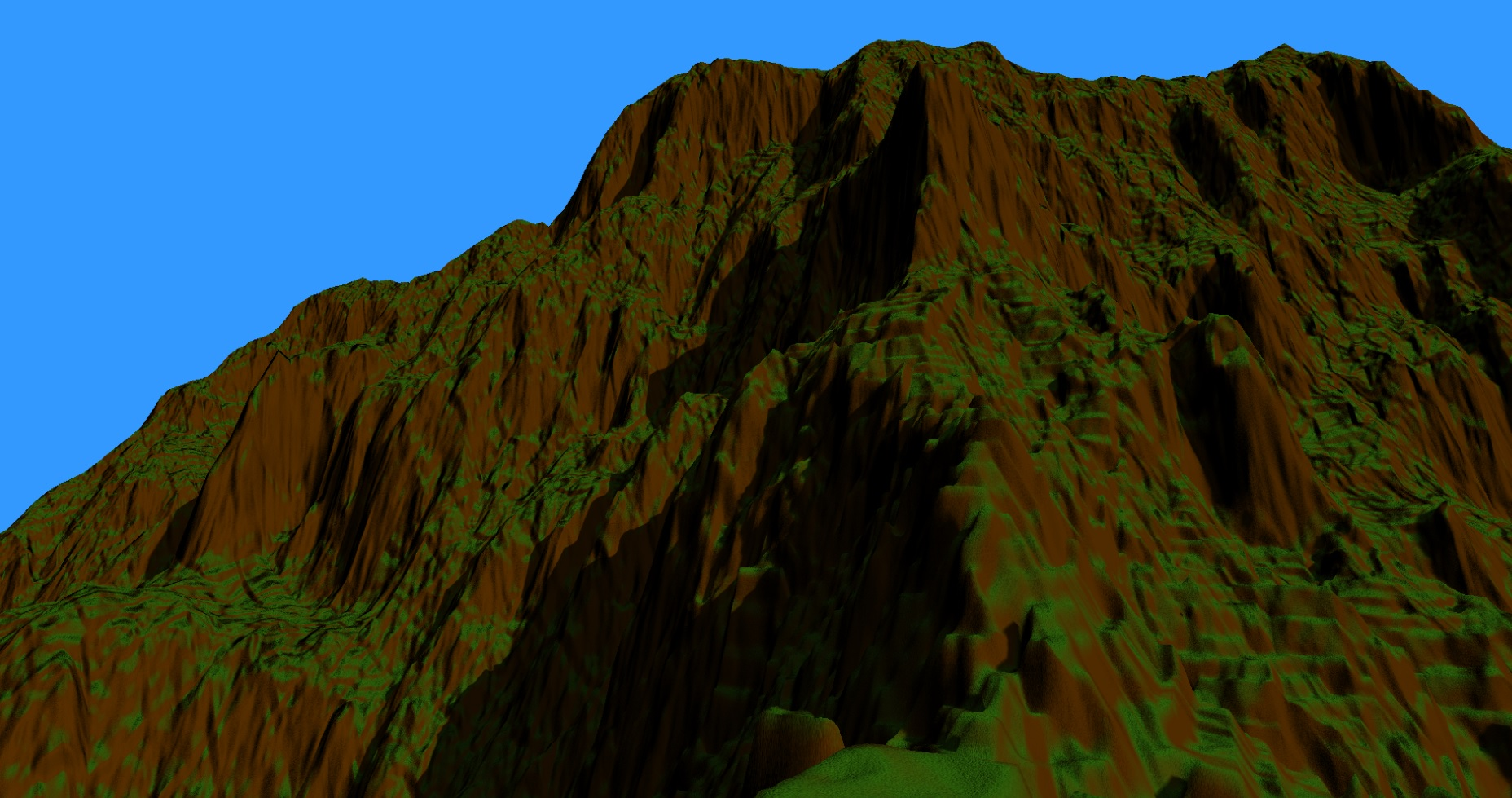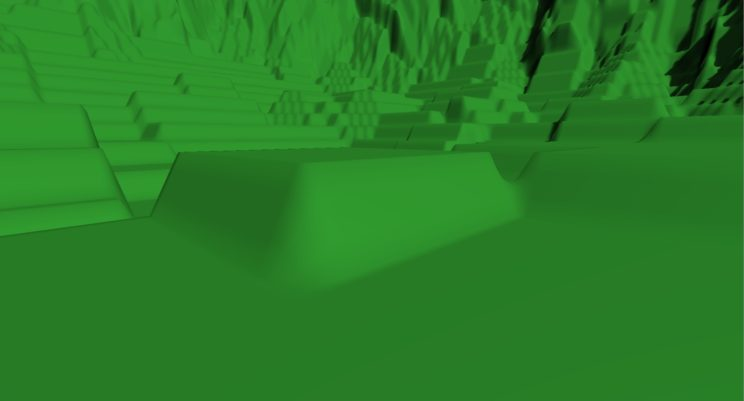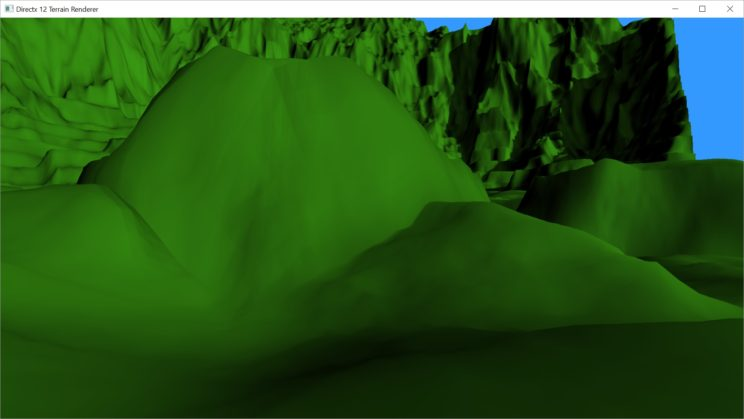# Rendering Terrain Part 22 – A Quick Fix For AABB

Last post I mentioned that there was an intermittent problem with view frustum culling caused by the displacement mapping. Occasionally, patches around the edges of the screen would get culled early as you’re looking or moving around. It didn’t happen often, so it was hard to spot, but the problem is obvious when you think about what’s happening.
Each quad patch used to define the initial mesh has an Axis Aligned Bounding Box (AABB) associated with it. In Part 10, we defined the AABB as the (x, y) positions of the bottom left and top right control points, and the minimum and maximum height values from the height map across that entire region. We also expanded the region by one in each direction when finding the height values to account for linear interpolation of values in the tessellation stages.

```// calculate the minimum and maximum z values for vertices between the provided bounds.
XMFLOAT2 Terrain::CalcZBounds(Vertex bottomLeft, Vertex topRight) {
float max = -100000;
float min = 100000;
int bottomLeftX = bottomLeft.position.x == 0 ? (int)bottomLeft.position.x: (int)bottomLeft.position.x - 1;
int bottomLeftY = bottomLeft.position.y == 0 ? (int)bottomLeft.position.y : (int)bottomLeft.position.y - 1;
int topRightX = topRight.position.x >= mWidth ? (int)topRight.position.x : (int)topRight.position.x + 1;
int topRightY = topRight.position.y >= mWidth ? (int)topRight.position.y : (int)topRight.position.y + 1;

for (int y = bottomLeftY; y <= topRightY; ++y) {
for (int x = bottomLeftX; x <= topRightX; ++x) {
float z = maImage[x + y * mWidth] * mHeightScale;

if (z > max) max = z;
if (z < min) min = z;
}
}

return XMFLOAT2(min, max);
}```

This was fine for just one height map, but now that we’re using a second height map as a displacement map, that displacement moves the vertices so that many are outside of that initial AABB.
We went from this:To this:That’s a pretty significant change. But what it actually amounts to isn’t all that much. Here’s the displacement code from our Domain Shader:

```float3 norm = estimateNormal(output.worldpos / width);
output.worldpos += norm * (displacementmap.SampleLevel(displacementsampler, output.worldpos / 32, 0.0f).w - 0.5f);```

So all we actually do is calculate the normal at the vertex before displacement, sample the displacement map, multiply the two numbers together, and add the result to the position of the vertex.
Since our normal is a unit vector, no component of the (x, y, z) normal vector will be less than -1 or greater than 1. Further, all values in our displacement map are in the range [0, 1]. We then subtract 0.5 from that, putting our displacement value in the range [-0.5, 0.5]. Thus, we are guaranteed to never be adding more than 0.5 to any x, y, or z component of any vertex.
So our quick fix is just to subtract 0.5 from the components of the minimum and add 0.5 to the maximums for our AABB. With that done, I cannot detect any further issues with the View Frustum Culling.
Of course, if we change the scaling on the displacement mapping, this would change the offsets for the AABBs. Right now, it is hard-coded to always be a scale of one. I may change that in the future, but it would be a matter of just applying the same scale to the offsets.

This is by far the shorted post so far in this series. Hey, apparently I can’t write an essay on every topic! That’s gotta be a good thing, right?
I was going to start talking about slope based colouring and texturing, but I haven’t figured out how to blend between two or more separate normal maps just yet. My goal is to have a different normal map for different materials, so if we have grass for flat areas and rock for vertical regions, they’ll actually have different normal maps applied and blend together correctly. So far, that isn’t really going well. If I don’t have that figured out by Friday, then the next post will just look at colouring the terrain. That, at least, was reasonably easy and doesn’t look too bad.
The featured image today shows what things look like so far. There is normal mapping, it just isn’t blending correctly. And I’ve only got slope based colouring so far. I also want height based. My goal is four different colours and textures: grass, dirt, rock, and snow.

For the latest version of the code, see GitHub.

Traagen